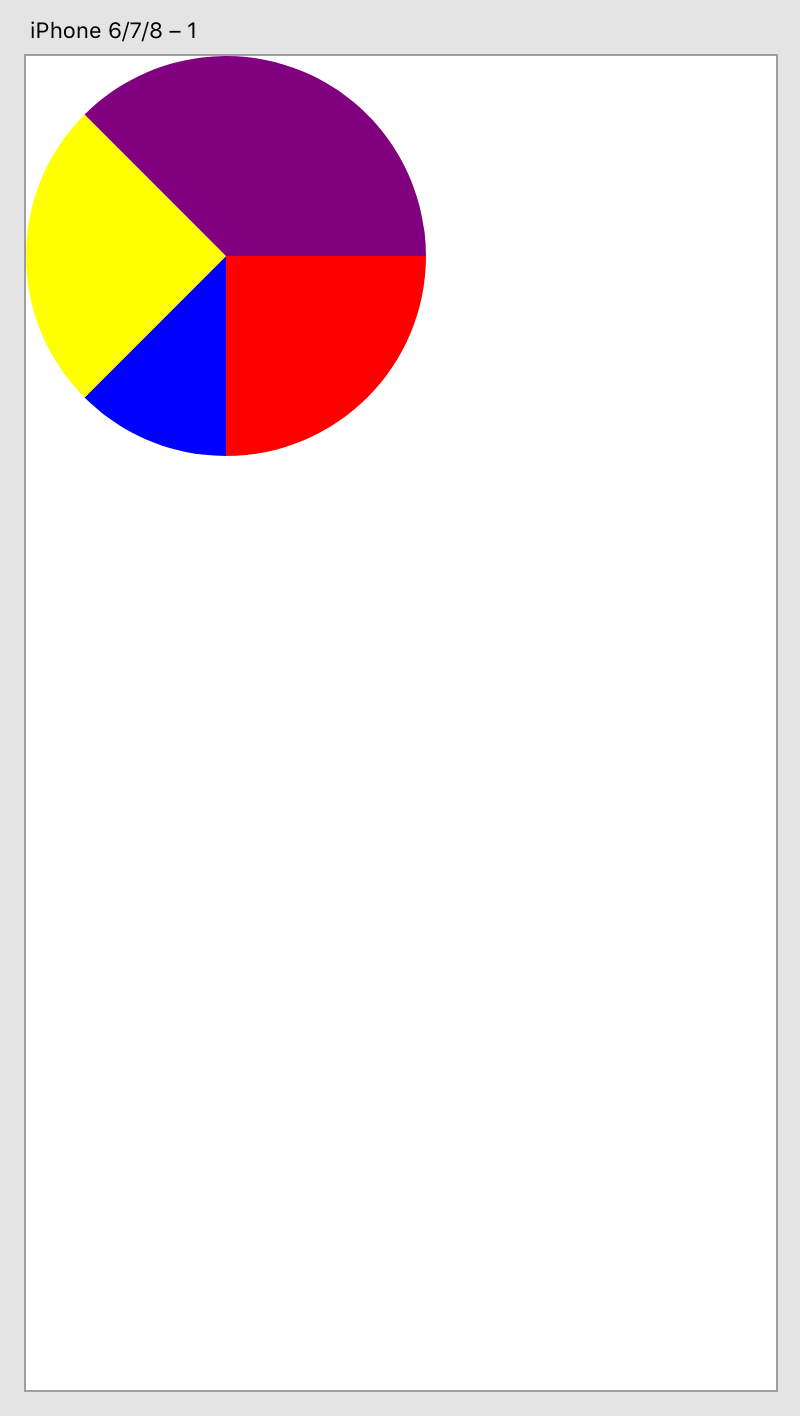# How to create paths

This sample demonstrates how to create path objects in XD. The path objects are used to construct a pie chart.

## Development Steps

Complete code for this plugin can be found on GitHub.

### 1. Create plugin scaffold

First, edit the manifest file for the plugin you created in our Quick Start Tutorial.

Replace the `uiEntryPoints` field of the manifest with the following:

``````"uiEntryPoints": [
{
"type": "menu",
"label": "Create Pie Chart",
"commandId": "createPieChartCommand"
}
]
``````

If you're curious about what each entry means, see the manifest documentation, where you can also learn about all manifest requirements for a plugin to be published in the XD Plugin Manager.

Then, update your `main.js` file, mapping the manifest's `commandId` to a handler function.

Replace the content of your `main.js` file with the following code:

``````function createPieChartCommand(selection) {
// The body of this function is added later
}

module.exports = {
commands: {
createPieChartCommand
}
};
``````

The remaining steps in this tutorial describe additional edits to the `main.js` file.

### 2. Require in XD API dependencies

For this tutorial, we just need access to two XD scenegraph classes.

Add the following lines to the top of your `main.js` file:

``````// Add this to the top of your main.js file
const { Path, Color } = require("scenegraph");
``````

Now the `Path` and `Color` classes are required in and ready to be used.

### 3. Create helper functions

##### Calculate a point on a circle

Since our plugin will create a pie chart, this helper function will be used to return an x,y coordinates of each wedge in the pie chart. The function accepts `radius` and `angle` as parameters and use them to calculate the coordinates of a point on a circle at a given angle on the perimeter of the circle.

Add the lines of code below to your file:

``````function pointOnCircle(radius, angle) {
const radians = angle * 2. * Math.PI / 360.;
const xcoord = radius * Math.cos(radians);
const ycoord = radius * Math.sin(radians);
return xcoord + "," + ycoord;
}
``````

This is just straight-up JavaScript; there's nothing specific to XD plugin APIs to cover here.

We won't cover the math in depth, here's an overview what this helper function does:

• The angle is expressed in degrees. It must be converted to radians before passing it to the sine and cosine functions. More info: Math.cos, Math.sin
• The function will return the coordinates as a string in the `x,y` format, which will be used to be inserted to the path data, which also has to be a string
##### Add a single pie wedge to the scenegraph

Our plugin will also need to be able to add a single pie wedge to the scenegraph.

Add the lines of code below to your file. Each of the numbered comments are explained below the code:

``````function createWedge(selection, radius, startAngle, endAngle, color) { // 
const startPt = pointOnCircle(radius, startAngle);
const endPt = pointOnCircle(radius, endAngle);
const pathData = `M0,0 L\${startPt} A\${radius},\${radius},0,0,1,\${endPt} L0,0`; // 
const wedge = new Path(); // 
wedge.pathData = pathData; // 
wedge.fill = new Color(color); // 
wedge.translation = {x: radius, y: radius};  // 
selection.insertionParent.addChild(wedge); // 
}
``````
1. This function accepts five arguments:
1. The current selection in the scene graph (`selection`)
2. The pie chart radius (`chartRadius`)
3. The start radian of the wedge (`startAngle`)
4. The end radian of the wedge (`endAngle`)
5. The color of the wedge (`color`)
2. Based on these arguments, `pathData` is constructed. The pen is moved to the origin, a line is drawn to the first point on the edge of the circle, an arc is drawn to the second point on the edge of the circle, and then a line is drawn back to the origin. For more information on how to create path data, please refer to Paths
3. Create a new instance of `Path`
4. Set `pathData`
5. Set the color of the path object
6. Move the path object down and to the right by `radius` units. As a result, the pie chart will appear with its top left corner positioned at `0,0`
7. Insert the path object into the currently-selected artboard

### 4. Create the main function

In this step, we'll build out the main function, `createLinesHandlerFunction`, that we added in the first step.

This function creates four wedges:

``````function createPieChartCommand(selection) {
createWedge(selection, 100, 0, 90, "red");
createWedge(selection, 100, 90, 135, "blue");
createWedge(selection, 100, 135, 225, "yellow");
createWedge(selection, 100, 225, 360, "purple");
}
``````

Note that the end angle of each wedge matches the start angle of the next wedge. As a result, the wedges fit together to create a complete pie chart!

### 5. Run the plugin

Ater saving all your changes, reload the plugin in XD and run it. The result should be similar to the following:Figure: Created pie chart

## Next Steps

Want to expand on what you learned here? Have a look at these references to see options for customizing this sample plugin:

Ready to explore further? Take a look at our other resources: